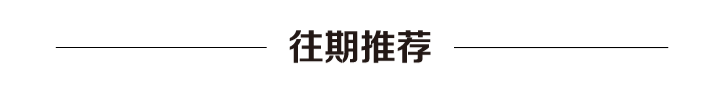### 题目描述

输入: [1,2,3]   1  /  2   3输出: 6

输入: [-10,9,20,null,null,15,7]   -10   /   9  20    /     15   7输出: 42

### 题目分析

• root 和左右路径形成路径（left – root – right）

• root 和左路径形成路径（left – root）

• root 和右路径形成路径（root – right）

• root 自成路径（root）

### 代码实现

private int maximum = Integer.MIN_VALUE;public int maxPathSum(TreeNode root) {    if (root == null) {        return 0;    }    helper(root);    return maximum;}private int helper(TreeNode root) {    if (root == null) {        return 0;    }    // 如果左右子树返回的最大路径值小于 0    // 直接将值设为 0，也就是不考虑对应的路径    int leftMax = Math.max(0, helper(root.left));    int rightMax = Math.max(0, helper(root.right));    //自底向上的分治,直到到了最底层，才开始计算并返回答案    maximum = Math.max(root.val + leftMax + rightMax, maximum);    return Math.max(leftMax + root.val, rightMax + root.val);}—  —